# 利用运动学实现导弹仿真飞行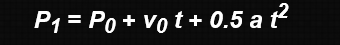P1是最后的位置，P0是初始位置，v0初始速度，a加速度，t是运动持续时间。我们在纸上推导一下数学公式，看能到什么结果？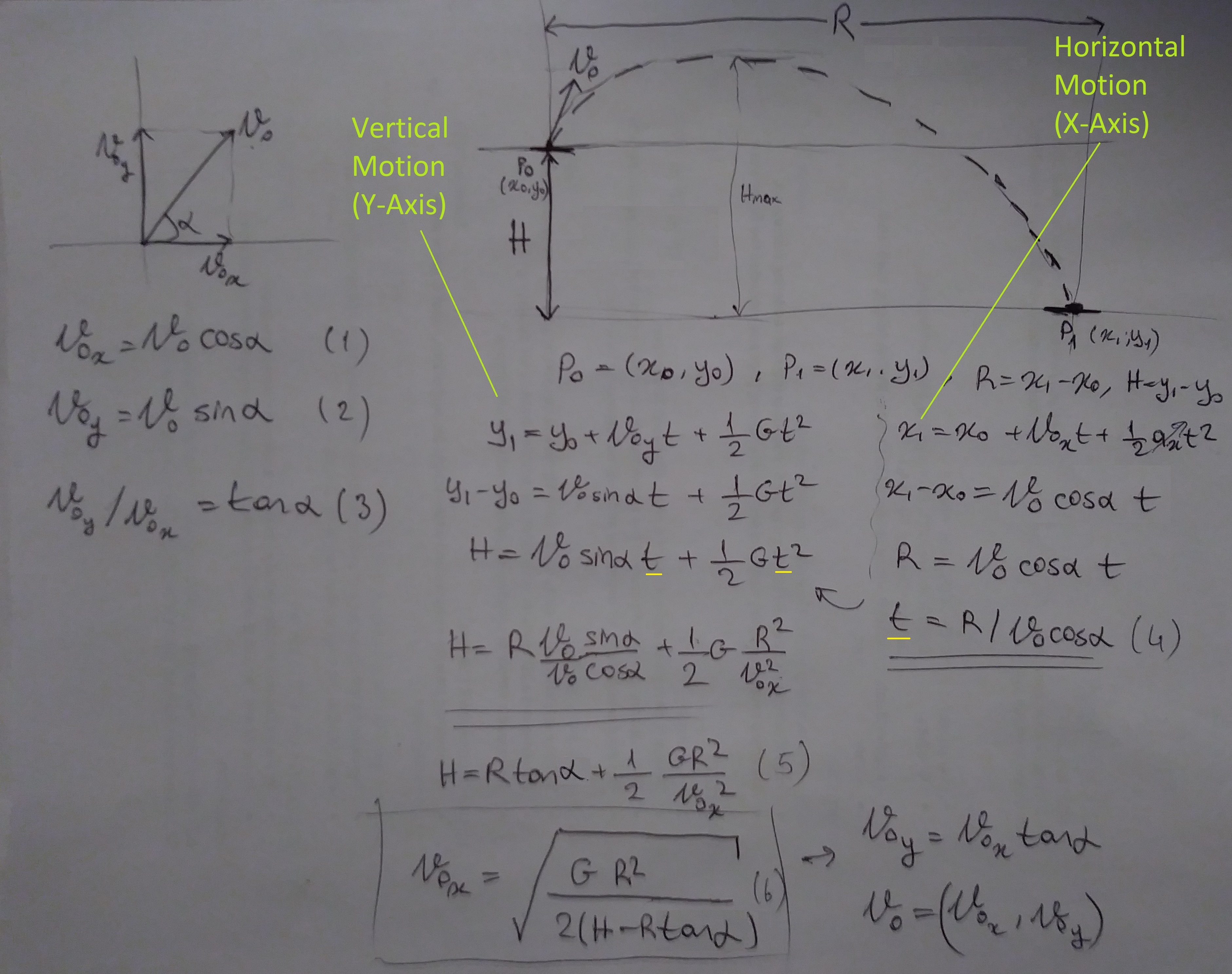a是发射角度，V0是初始速度，V0x，V0y是初始速度V0的两个分量，H是沿着y轴位置距离差，R是沿x轴的位置距离差，t就是抛物线运动的时间。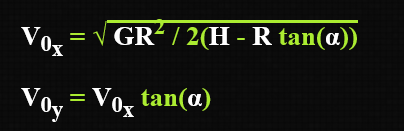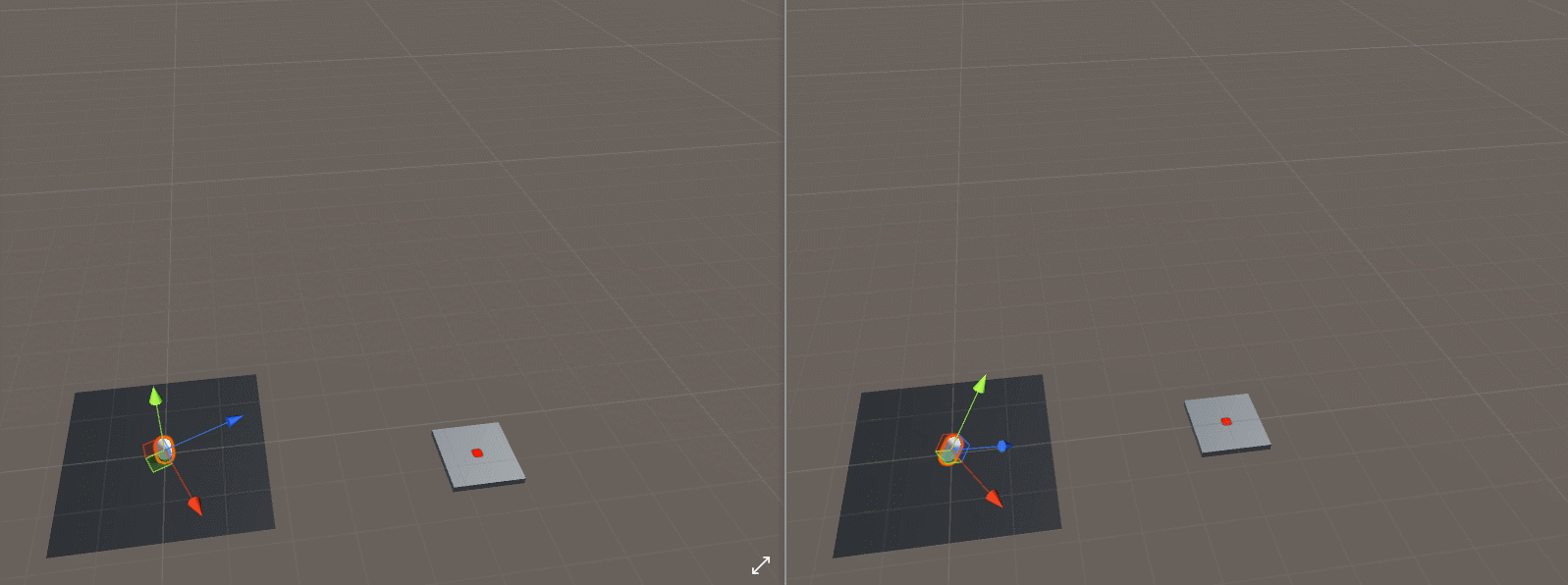void Launch()
{
// think of it as top-down view of vectors:
//   we don't care about the y-component(height) of the initial and target position.
Vector3 projectileXZPos = new Vector3(transform.position.x, 0.0f, transform.position.z);
Vector3 targetXZPos = new Vector3(TargetObjectTF.position.x, 0.0f, TargetObjectTF.position.z);

// rotate the object to face the target
transform.LookAt(targetXZPos);

// ...
}


R = Vector3.Distance(projectileXZPos, targetXZPos);
H=TargetObjectTF.position.y - transform.position.y;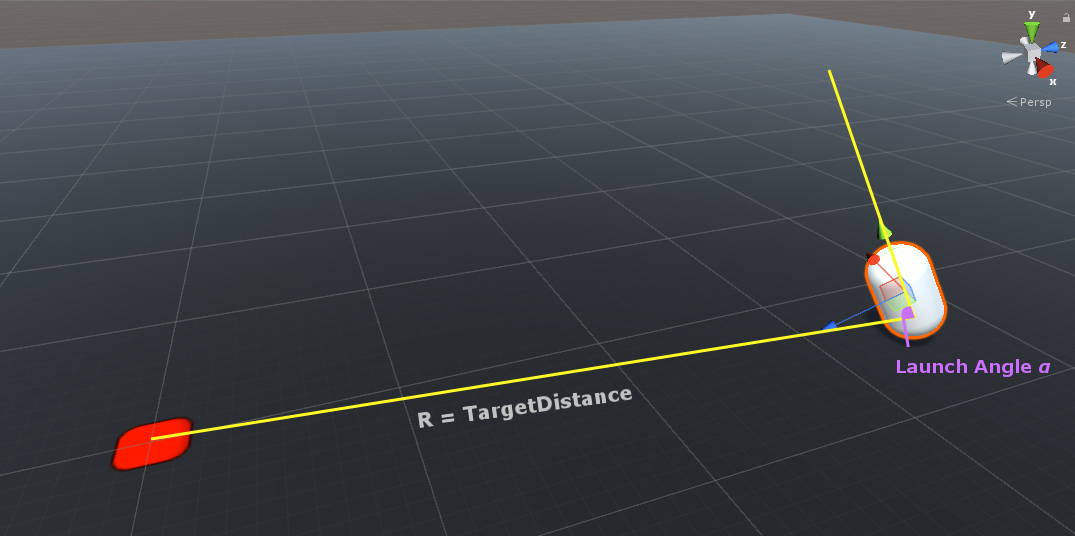公式计算出来后，我们要将公式用编程语言表达出来，这个也就是所谓的理论联系实际，对应代码如下所示：

void Launch()
{
// ...

// shorthands for the formula
float R = Vector3.Distance(projectileXZPos, targetXZPos);
float G = Physics.gravity.y;
float tanAlpha = Mathf.Tan(LaunchAngle * Mathf.Deg2Rad);
float H = (TargetObjectTF.position.y + GetPlatformOffset()) - transform.position.y;

// calculate initial speed required to land the projectile on the target object
float Vz = Mathf.Sqrt(G * R * R / (2.0f * (H - R * tanAlpha)) );
float Vy = tanAlpha * Vz;

// create the velocity vector in local space and get it in global space
Vector3 localVelocity = new Vector3(0f, Vy, Vz);

// ...
}


void Launch()
{
// think of it as top-down view of vectors:
//   we don't care about the y-component(height) of the initial and target position.
Vector3 projectileXZPos = new Vector3(transform.position.x, 0.0f, transform.position.z);
Vector3 targetXZPos = new Vector3(TargetObjectTF.position.x, 0.0f, TargetObjectTF.position.z);

// rotate the object to face the target
transform.LookAt(targetXZPos);

// shorthands for the formula
float R = Vector3.Distance(projectileXZPos, targetXZPos);
float G = Physics.gravity.y;
float tanAlpha = Mathf.Tan(LaunchAngle * Mathf.Deg2Rad);
float H = TargetObjectTF.position.y - transform.position.y;

// calculate the local space components of the velocity
// required to land the projectile on the target object
float Vz = Mathf.Sqrt(G * R * R / (2.0f * (H - R * tanAlpha)) );
float Vy = tanAlpha * Vz;

// create the velocity vector in local space and get it in global space
Vector3 localVelocity = new Vector3(0f, Vy, Vz);
Vector3 globalVelocity = transform.TransformDirection(localVelocity);

// launch the object by setting its initial velocity and flipping its state
rigid.velocity = globalVelocity;
}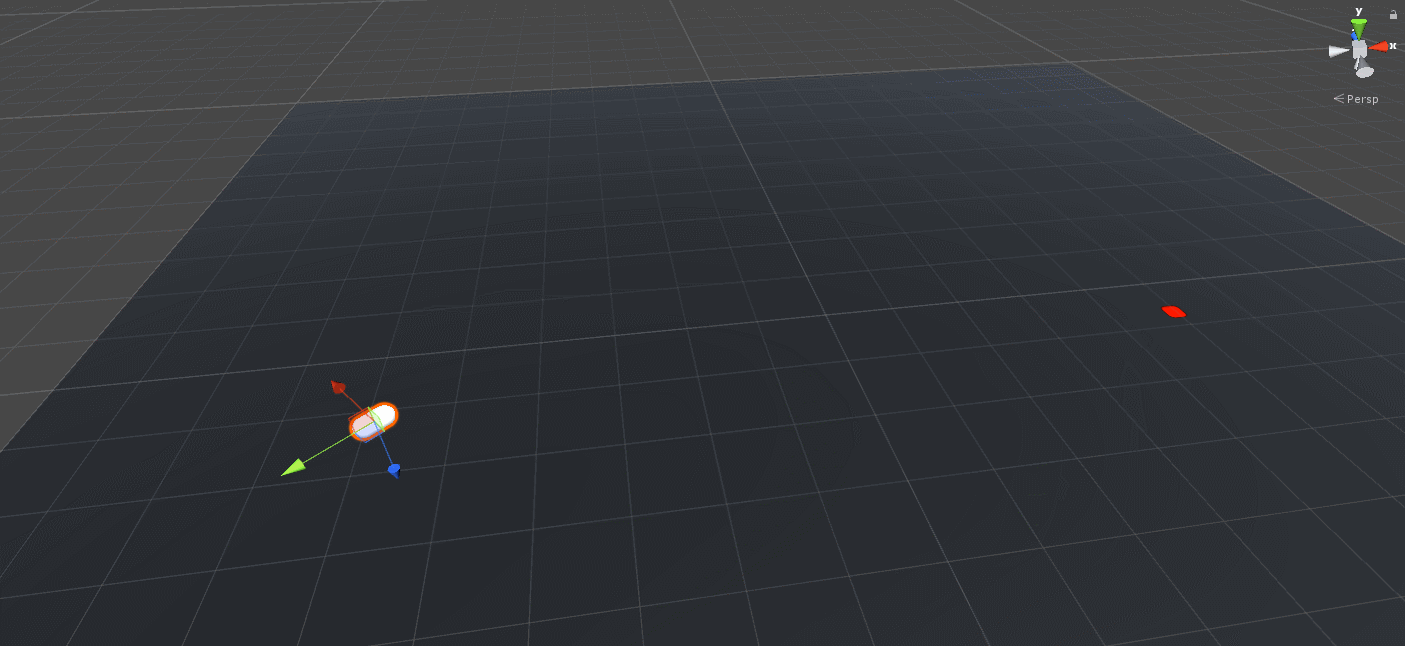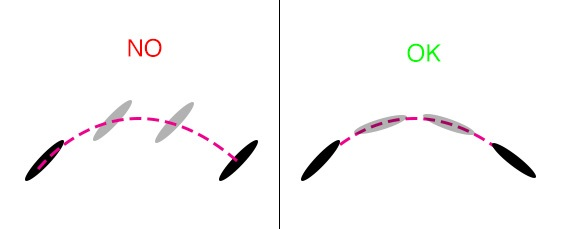public class Trajectory : MonoBehaviour
{
//...

// state
private bool bTouchingGround;

//...

// Update is called once per frame
void Update ()
{
// ...

{
// update the rotation of the projectile during trajectory motion
transform.rotation = Quaternion.LookRotation(rigid.velocity);
}
}

void OnCollisionEnter()
{
bTouchingGround = true;
}

void OnCollisionExit()
{
bTouchingGround = false;
}
}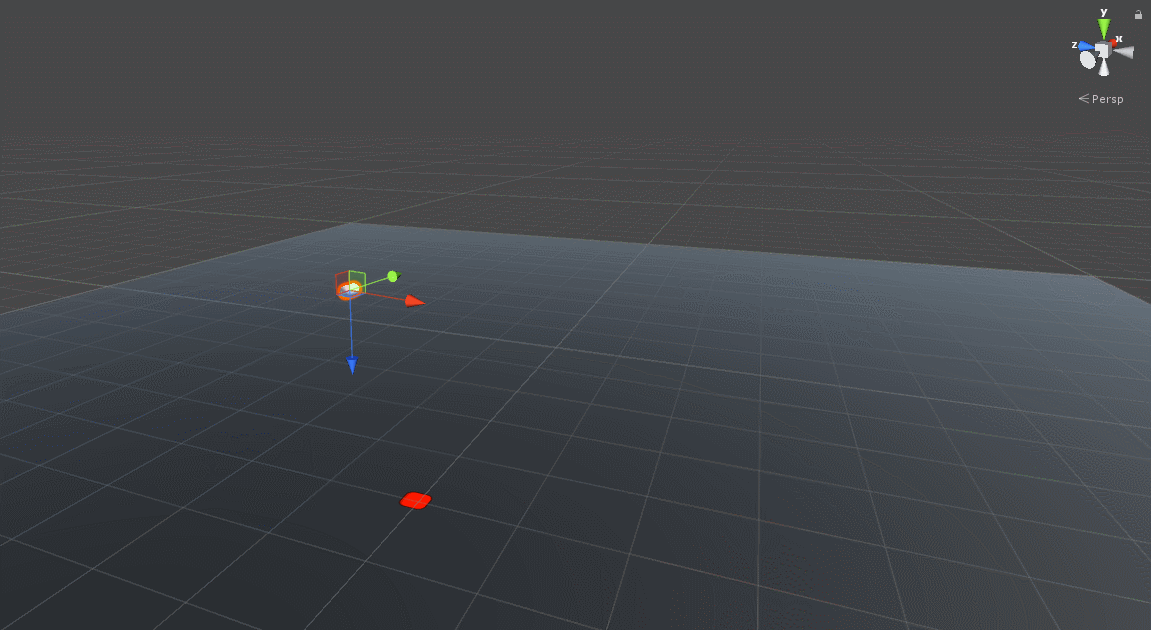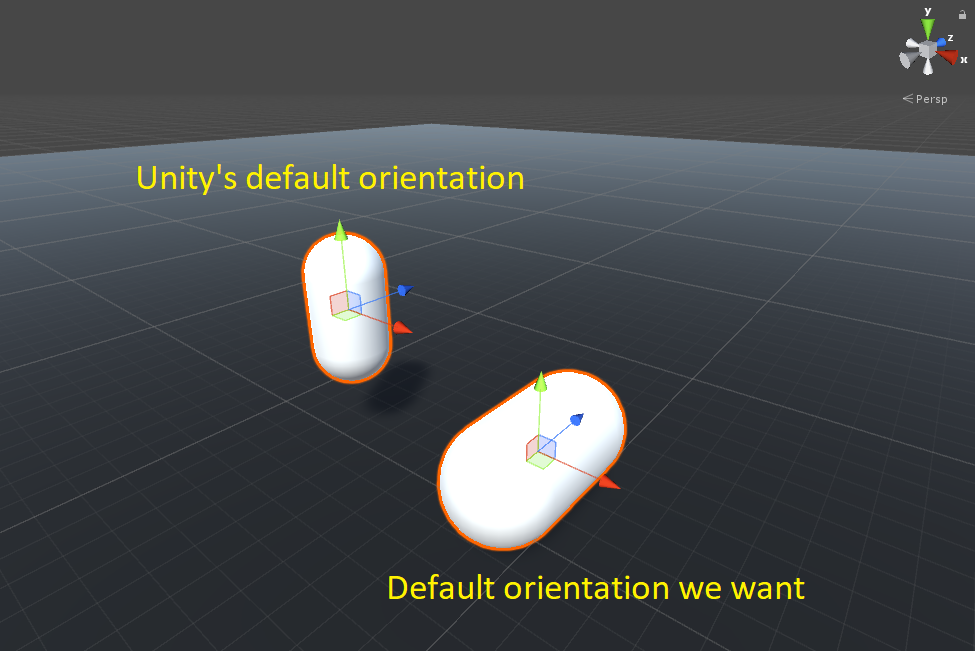transform.rotation = Quaternion.LookRotation(rigid.velocity) * initialRotation;


https://math.stackexchange.com/questions/331539/combining-rotation-quaternions/331548#331548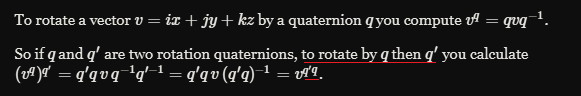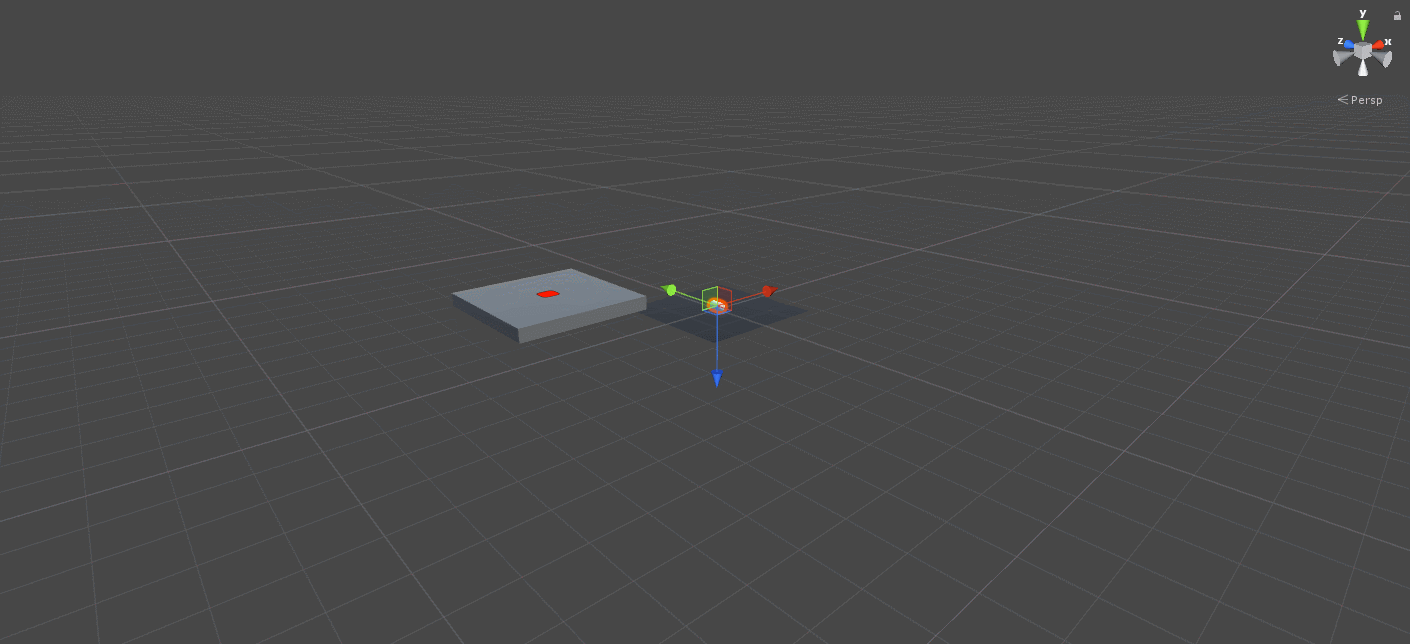demo下载地址：链接：https://pan.baidu.com/s/1-3R9HN045xVJlAejTjl7gQ05-07
07-298129
04-23679
07-01
03-255748
09-14
12-132万+
09-02
05-31